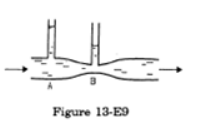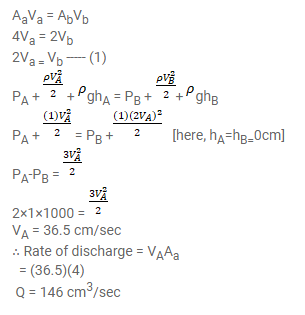# Water flows through a horizontal tube as shown in figure (13-E9).

Question:

Water flows through a horizontal tube as shown in figure (13-E9). If the difference of heights of water column in the vertical tubes is $2 \mathrm{~cm}$, and the areas of cross-section at $A$ and $B$ are $4 \mathrm{~cm}^{2}$ and $2 \mathrm{~cm}^{2}$ respectively, find the rate of flow of water across any section.Solution: